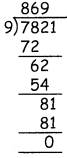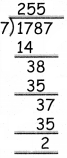Tamilnadu State Board New Syllabus Samacheer Kalvi 4th Maths Guide Pdf Term 3 Chapter 2 Numbers Ex 2.2 Textbook Questions and Answers, Notes.

## Tamilnadu Samacheer Kalvi 4th Maths Solutions Term 3 Chapter 2 Numbers Ex 2.2

Divide the following

Question 1.
5632 ÷ 6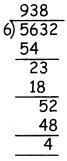Quotient = 938
Remainder = 4Question 2.
7460 ÷7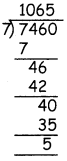Quotient = 1065
Remainder = 5

Question 3.
4964 ÷ 8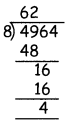Quotient = 62
Remainder = 4Question 4.
8616 ÷ 6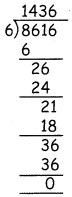Quotient = 1436
Remainder = 0

Question 5.
8645 ÷ 7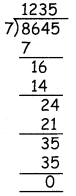Quotient = 1235
Remainder = 0Question 6.
5742 ÷ 9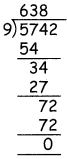Quotient = 638
Remainder = 0

Question 7.
In my school, there are 1115 students from class 1 to 8.
If the number of students is same in all the classes, how many
students are there in each class?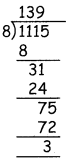139 studentsQuestion 8.
The height of a mountain is 7821 m. Raj took 9 days to reach the top.
How many metres did he cover daily if he travelled equal distances every day?